## STAM 102 :: Lecture 09 :: Use of in-built functions and writing expressions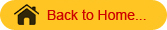# In-built Functions

• A function is an in-built program, which is used to do a particular task.
• Functions take the input the input and will give the result as the output.
• Based on the input and output data the functions are categorized as
• String functions
• Arithmetic functions
• Date functions
• Logical functions
• Group functions

Use of in-built function SUM()
To use in-built functions enter the data to prepare mark list of the I- B.Sc.(Agriculture) students in the spreadsheet.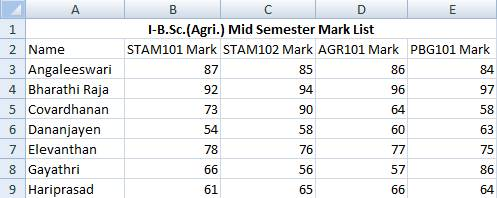• Using the in-built function SUM() we can calculate the total scored by each and every student in I-B. Sc. (Agri.).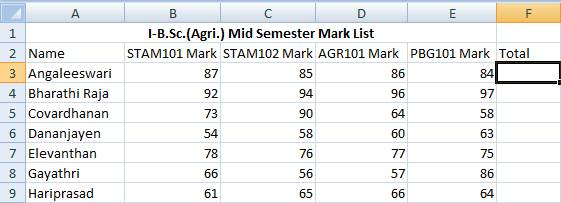• Place the mouse pointer in the cell with the address F3
• The F3 cell is the one which should display the total mark scored by the student namely Angaleeswari in the above example.
• Click on Insert Menu à Function
• or Select fx in the Formula bar
• Insert Function dialog box will get displayed as shown below: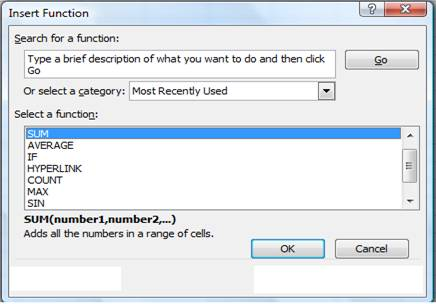• Select SUM function and click OK button in the Insert Function dialog box.
• The function Argument dialog box will be displayed with the automatically assumed range of cells to be added(B3 to E3)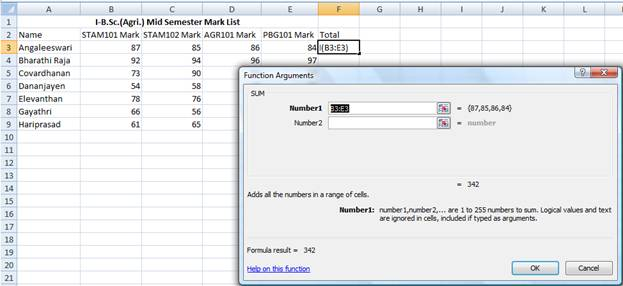• Click OK. The result is displayed as shown. We can even choose the range of cells added manually.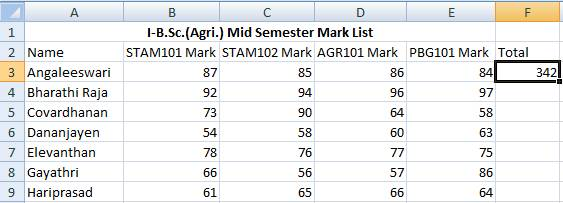• The total marks scored by the other students have to added in the same way by making use of the SUM() function.
• Instead entering the same function for all the students in the example we can copy the formula to the cells in the total column to add B4:E4, B5:E5, B6:E6 and so on.
• When we copy the SUM() function formula from the cell F3 to F4 the SUM function will automatically taking the input range of numbers to be added is B4:E4.
• The same is applicable to the rest of the cells in the total column.
• To copy down the formula place the mouse pointer at the bottom right corner of the cell F3.
• The mouse pointer now automatically changes into + symbol.
• Now drag + symbol down the cells in the Total column.
• We can see the total marks of all the students in the example as shown below.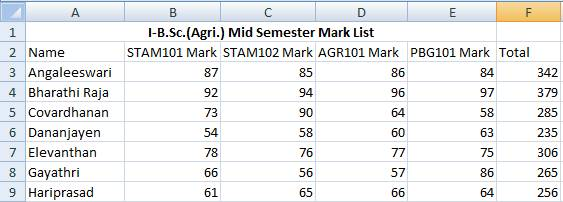Use of in-built function AVERAGE()

• To calculate the average marks scored by the students in the example we can make use of average function AVERAGE().
• Enter Average column in the spreadsheet.
• Place the mouse pointer in the cell with the address G3
• The F3 cell is the one which should display the total mark scored by the student namely Angaleeswari in the above example.
• Click on Insert Menu à Function
• or Select fx in the Formula bar
• Insert Function dialog box will get displayed as shown below. Choose AVERAGE() function and click OK.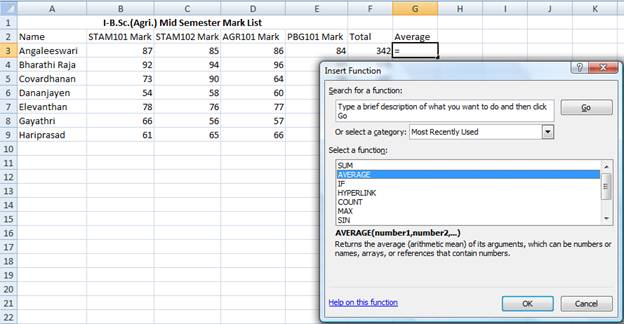• Click OK button in the Insert Function dialog box.
• In the Function Argument dialog box enter F3/4  then click OK button. The average scored is displayed.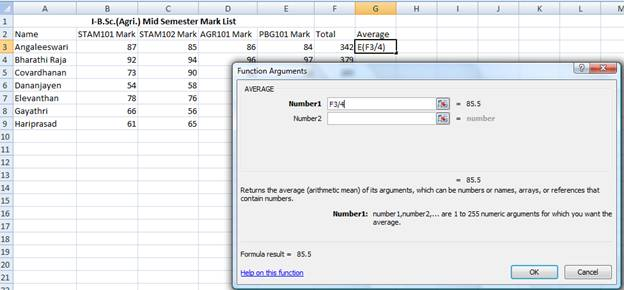• Copy the AVERAGE formula as we copied the SUM().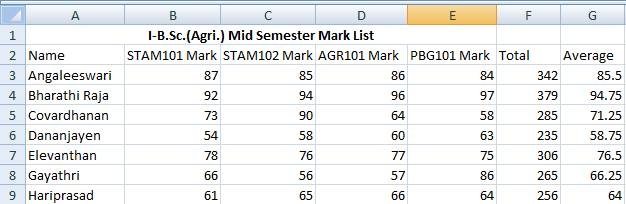# Writing Expressions

• The total marks can be calculated by writing expressions.
• Place the cursor the cell F3.
• To enter expression, enter the equal sign first.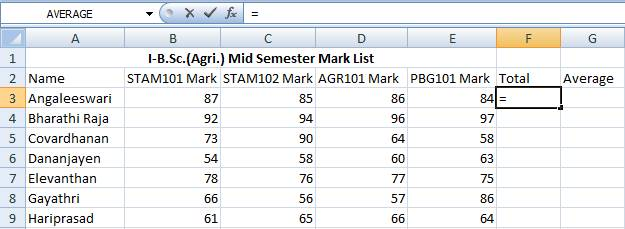• Choose the cells with the cursor as sown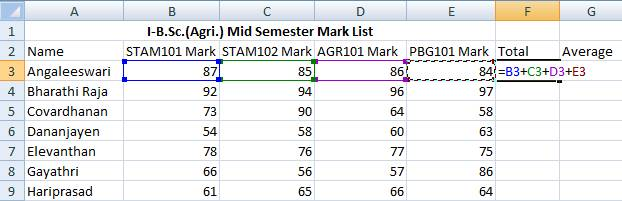• Press enter. The result will be displayed in F3. Copy the expression down the Total column to find the total mark scored by all the students in the example.
• To calculate the averages place the G3.
• Enter the equal sign first in the cell G3.
• Enter F3/4 which is the average to be calculated.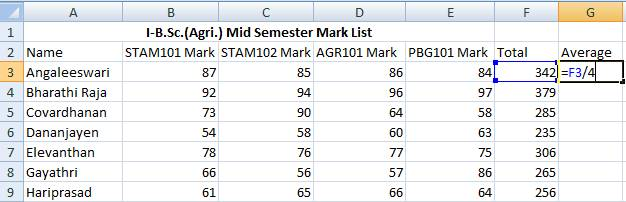• Press enter. The average will be displayed. Copy the expression down the cells in the Average column to calculate the rest of the averages.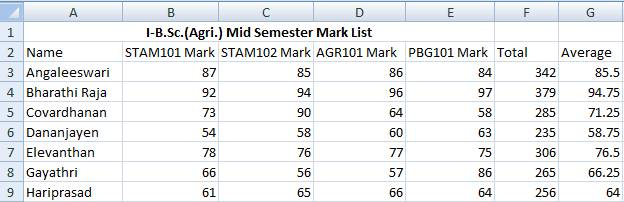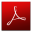Download this lecture as PDF here
`